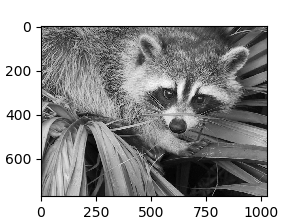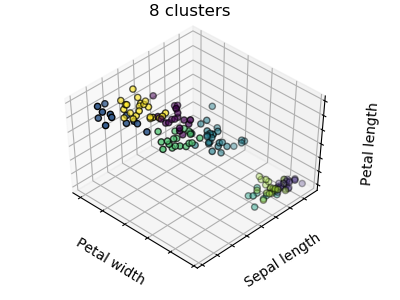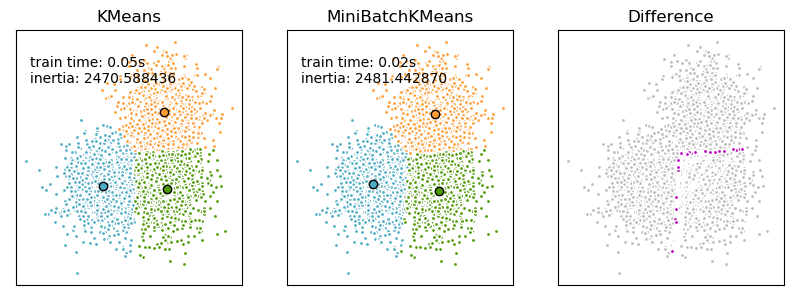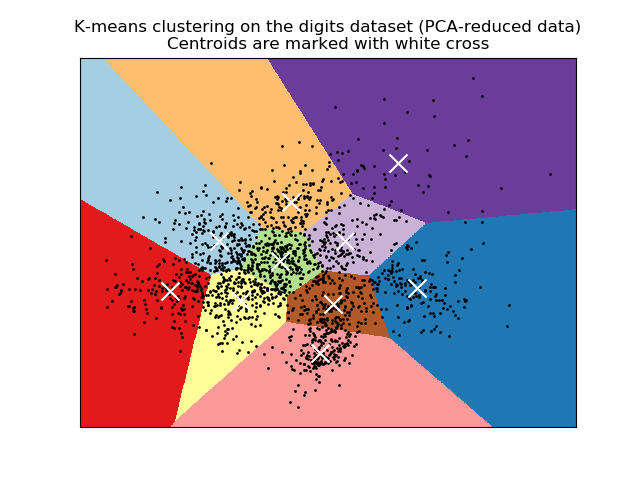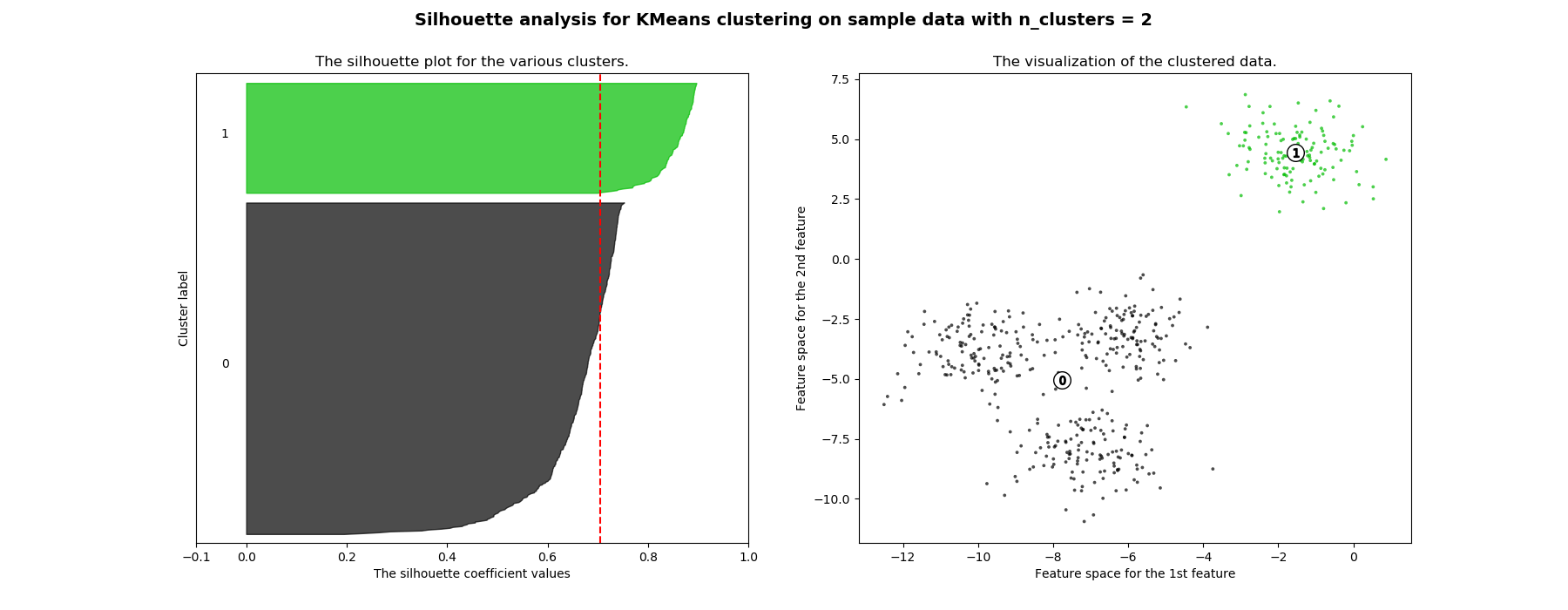# sklearn.cluster.KMeans¶

class sklearn.cluster.KMeans(n_clusters=8, *, init='k-means++', n_init=10, max_iter=300, tol=0.0001, precompute_distances='deprecated', verbose=0, random_state=None, copy_x=True, n_jobs='deprecated', algorithm='auto')

[源码]

K-均值聚类

n_clusters int, default=8

init {‘k-means++’, ‘random’, ndarray, callable}, default=’k-means++’

‘k-means++’：明智地选择初始聚类中心进行k均值聚类，加快收敛速度.有关详细信息，请参阅k_init中的Notes部分。
‘random’：从初始质心的数据中随机选择n_clusters观测(行)。

max_iter int, default=300

precompute_distances {‘auto’, True, False}, default=’auto’

‘auto’：如果n个样本n_samples * n_clusters > 1200万，不预先计算距离。这相当于使用双精度的每个作业大约100 MB的开销。
True :总是预先计算距离
False：永远不要预先计算距离。

verbose int, default=0

random_state int, RandomState instance, default=None

copy_x bool, default=True

n_jobs int, default=None

None-1意味着使用所有处理器。

algorithm {“auto”, “full”, “elkan”}, default=”auto”

*在版本0.18中更改：*添加了Elkan算法

cluster_centers_ ndarray of shape (n_clusters, n_features)

labels_ ndarray of shape (n_samples,)

inertia_ float

n_iter_ int

• MiniBatchKMeans

替代性在线实施，使用迷你批次对中心位置进行增量更新。对于大规模学习（例如n_samples> 10k），MiniBatchKMeans可能比默认的批处理实现要快得多。

k-均值问题采用Lloyd’s算法或Elkan’s算法求解。

>>> from sklearn.cluster import KMeans>>> import numpy as np>>> X = np.array([[1, 2], [1, 4], [1, 0],...               [10, 2], [10, 4], [10, 0]])>>> kmeans = KMeans(n_clusters=2, random_state=0).fit(X)>>> kmeans.labels_array([1, 1, 1, 0, 0, 0], dtype=int32)>>> kmeans.predict([[0, 0], [12, 3]])array([1, 0], dtype=int32)>>> kmeans.cluster_centers_array([[10.,  2.],       [ 1.,  2.]])

fit(self, X[, y, sample_weight]) 计算k-均值聚类
fit_predict(self, X[, y, sample_weight]) 计算聚类中心并预测每个样本的聚类索引
fit_transform(self, X[, y, sample_weight]) 计算聚类并将X变换成簇距离空间
get_params(self[, deep]) 获取此估计器的参数
predict(self, X[, sample_weight]) 预测X中每个样本所属的最接近的聚类
set_params(self, **params) 设置此估计器的参数
transform(self, X) 将X转换为簇距离空间
__init__(self, n_clusters=8, *, init='k-means++', n_init=10, max_iter=300, tol=0.0001, precompute_distances='deprecated', verbose=0, random_state=None, copy_x=True, n_jobs='deprecated', algorithm='auto')

[源码]

fit(self, X, y=None, sample_weight=None)

[源码]

X {array-like, sparse matrix} of shape (n_samples, n_features), or (n_samples, n_samples)

y Ignored

sample_weight array-like of shape (n_samples,), default=None
X中每个观测值的权重，如果没有，则所有观测值都被赋予相同的权重。

self 拟合的估计器
fit_predict(self, X, y=None, sample_weight=None)

[源码]

X {array-like, sparse matrix} of shape (n_samples, n_features)

y Ignored 未使用，在此按约定呈现为API一致性。
sample_weight array-like of shape (n_samples,), default=None
X中每个观测值的权重，如果没有，则所有观测值都被赋予相同的权重。

labels ndarray of shape (n_samples,)

fit_transform(self, X, y=None, sample_weight=None)

[源码]

X {array-like, sparse matrix} of shape (n_samples, n_features)

y Ignored

sample_weight array-like of shape (n_samples,), default=None
X中每个观测值的权重，如果没有，则所有观测值都被赋予相同的权重。

labels array of shape (n_samples, n_clusters)
X在新空间中的变换
get_params(self, deep=True)

[源码]

deep bool, default=True

params mapping of string to any

predict(self, X, sample_weight=None)

[源码]

X {array-like, sparse matrix} of shape (n_samples, n_features)

sample_weight array-like of shape (n_samples,), default=None
X中每个观测值的权重，如果没有，则所有观测值都被赋予相同的权重。

labels ndarray of shape (n_samples,)

score(self, X, y=None, sample_weight=None)

[源码]

K-均值目标上X值的相反

X {array-like, sparse matrix} of shape (n_samples, n_features)

y Ignored

sample_weight array-like of shape (n_samples,), default=None
X中每个观测值的权重，如果没有，则所有观测值都被赋予相同的权重。

score float

set_params(self, **params)

[源码]

**params dict

self object

transform(self, X)

[源码]

X {array-like, sparse matrix} of shape (n_samples, n_features)

labels array of shape (n_samples, n_clusters)
X在新空间中的变换

## sklearn.cluster.KMeans使用示例¶